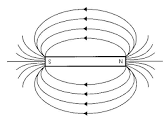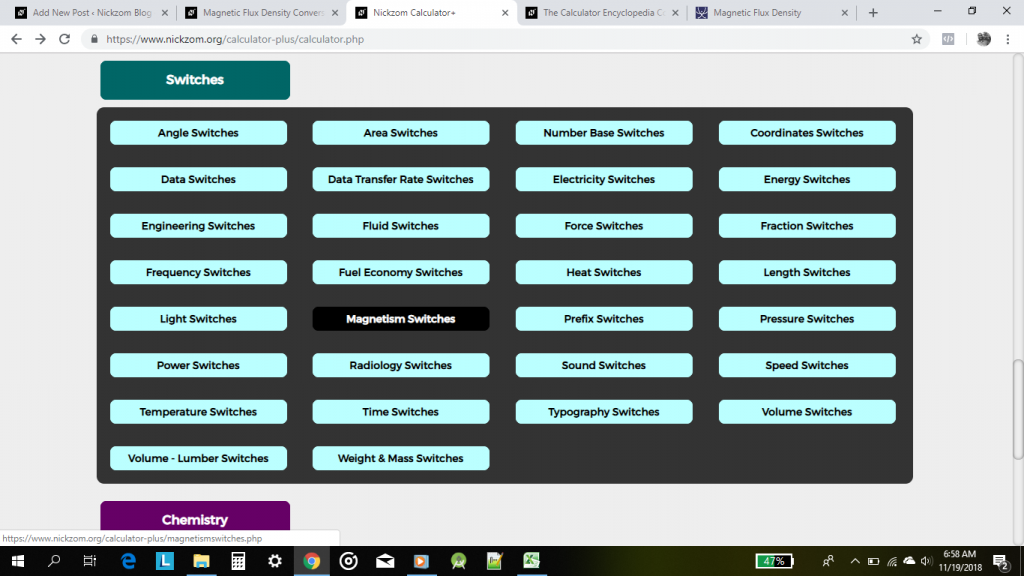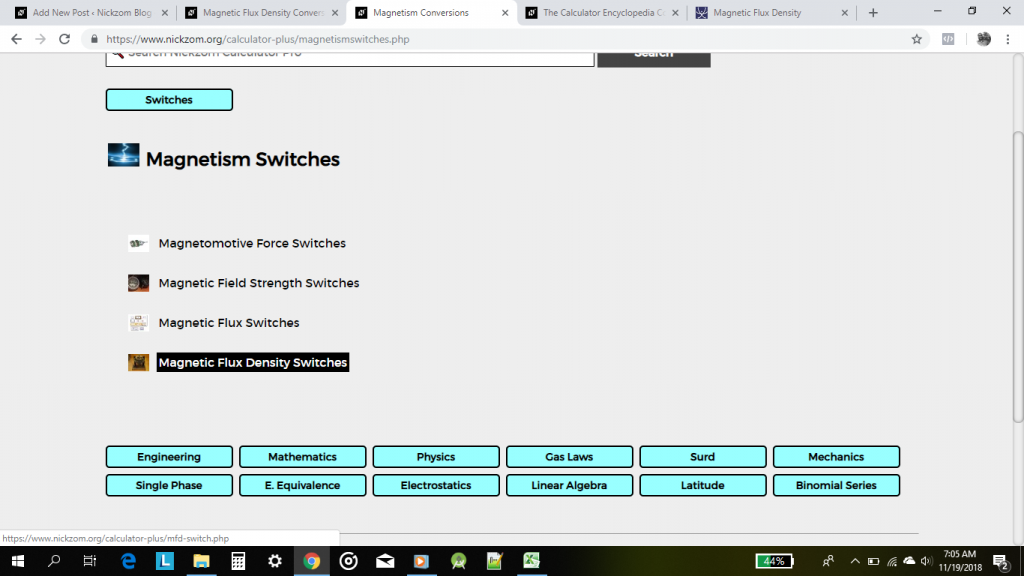## How to Calculate and Solve for Magnetic Flux Density or Field Induction | The Calculator EncyclopediaThe image above represents the magnetic flux density/field induction.

To compute the magnetic force of a field, four essential parameters are needed and the parameters are Magnetic Force (F), Quantity of Charge (q), Average Velocity of the Charge (v) and Angle between v and B (θ).

The formula for calculating the magnetic flux density/field induction:

B = F / qVsinθ

Where;
B = Magnetic Induction or Magnetic Flux Density
F = Magnetic Force
q = Quantity of Charge
v = Average Velocity of the charge
θ = Angle between v and B

Let’s solve an example;
Find the magnetic flux density/field induction of a field when Magnetic Force (F) is 17, Quantity of Charge (q) is 21, Average Velocity of the Charge (v) is 15 and Angle between v and B (θ) is 120°.

This implies that;
F = Magnetic Force = 17
q = Quantity of Charge = 21
v = Average Velocity of the charge = 15
θ = Angle between v and B = 120°

B = F / qVsinθ
B = 17 / (21 x 15)(sin 120°)
B = 17 / (315)(0.866)
B = 17 / (272.79)
B = 0.0623

Therefore, the magnetic flux density/field induction is 0.0623 Tesla.

## The Calculator Encyclopedia Converts Magnetic Flux Density Units on Magnetism Unit Conversions

According to Mini Physics,

Magnetic flux density (B) is defined as the force acting per unit current per unit length on a wire placed at right angles to the magnetic field.Nickzom Calculator converts 11 units of magnetic flux density such as:

• Tesla
• Weber/sq. meter
• Weber/sq. centimeter
• Weber/sq. inch
• Maxwell/sq. meter
• Maxwell/sq. centimeter
• Maxwell/sq. inch
• Gauss
• Lines/sq. centimeter
• Lines/sq. inch
• Gamma

On Clicking on Magnetism Switches, there are 4 major magnetism unit conversion sections such as:

• Magnetomotive Force Switches
• Magnetic Field Strength Switches
• Magnetic Flux Switches
• Magnetic Flux Density Switches